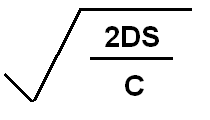In batch costing, we need to know what quantity of batch should be produced, so that our cost can be minimized. For this, we have to understand, what are main costs which affects units in a batch. There are two costs, first is setting cost per batch. This cost tells that if you start a match or plant for producing one batch, you have to pay for this. Second is carrying cost per unit. This cost is paid for keeping goods in store. On these both cost basis, we calculate economic batch quantity which shows that group of units in a batch. If we produce batches in these group of units, our overall cost will be minimum. That is the reason, we say it also optimum batch quantity or economic production quantity.

Formula of Economic Batch Quantity

D means =  Annual demand for production

S means = setup costs per batch

C means = inventory carrying cost  or storage cost per unit.

## \$type=three\$a=hide\$cm=hide\$s=hide\$show=/2019/06/10-steps-to-become-better-investor.html\$l=hide

Name

false
ltr
item
Accounting Education: Economic Batch Quantity
Economic Batch Quantity
http://1.bp.blogspot.com/_DJEIRrK4tl4/TNNT_YVX3gI/AAAAAAAAGGo/-uXCQFRu1GE/s1600/formula.PNG
http://1.bp.blogspot.com/_DJEIRrK4tl4/TNNT_YVX3gI/AAAAAAAAGGo/-uXCQFRu1GE/s72-c/formula.PNG
Accounting Education
http://www.svtuition.org/2010/11/economic-batch-quantity.html
http://www.svtuition.org/
http://www.svtuition.org/
http://www.svtuition.org/2010/11/economic-batch-quantity.html
true
2410664366776677676
UTF-8